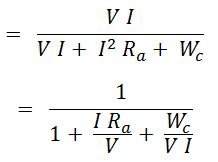# Efficiency of DC Generator & Condition for Maximum Efficiency

## Efficiency :

It may be defined as the ratio of output in watt (or KW) to the input in watt (or KW).

## Condition for Maximum Efficiency :

#### Let,Generator output = VI.Generator input = output + losses.= VI + Ia2 Ra + Wc= VI + I2 Ra + Wc(assuming, Ia = I ; since Ishis very small compared to Ia)Efficiency, η = power output / power input(By dividing Nr. and Dr. by VI)

To obtain the maximum efficiency the denominator should be minimum, therefore differentiating the denominator with respect to I and equate to zero.

#### Hence, the efficiency is maximum when,

VARIABLE LOSS = CONSTANT LOSS

Do not enter any spam links and messages